# Taylor Series for sin(x): How-to & Steps

Lesson Transcript
Instructor: Gerald Lemay

Gerald has taught engineering, math and science and has a doctorate in electrical engineering.

In this lesson, we show how to find the Taylor series for the sine function. Using examples, we look at the series evaluated about two different values of x.

## How to Find a Taylor Series

A Taylor series is an infinite series of terms. These terms have the form of a power of x multiplied by a coefficient. When the terms in the series are added together, we can approximate a function at a specific value of x, provided the value lies within the interval of convergence for the function. We won't show this, but the Taylor series for sin(x) works for all values of x.

We're going to find the Taylor series for sin(x) by using the general expression for the Taylor series. This will mean calculating various derivatives, substituting, and then simplifying.

Below is the general expression for the Taylor series of a function f(x):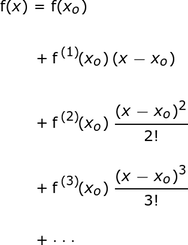That's quite a bit, though, isn't it? Here's a compact expression for the same sum of terms: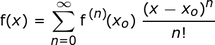In the expression above, xo is the value of x about which the series is calculated. The superscripts indicate derivatives. Thus, f (1) is the first derivative, f (2) is the second derivative, and so on. The Σ stands for ''sum'' and means we take values of n from 0 to ∞. We substitute these n's one at a time into the expression to the right of the Σ. Each of those terms are added together.

The exclamation point is the factorial, meaning:

2! = 2(1) = 2

3! = 3(2)(1) = 6

and so on.

In general,

n! = n(n-1)(n-2) … (1).

Also, 1! = 1 and 0! = 1.

Now let's go through the steps of finding the Taylor series for sin(x).

#### Step 1: Find the derivatives of f(x).

There's an infinite number of terms used in the summation. We will work out the first six terms in this list below.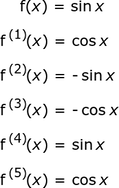It's important to note that, for the sine function, the derivatives repeat at every fourth differentiation. Thus, the fourth derivative of sin(x) is sin(x); the fifth derivative of sin(x) is the same as the first derivative, and so on.

#### Step 2: Evaluate these derivatives at xo

For now, we'll keep things general and simply substitute xo for x in the derivatives, which you can see here: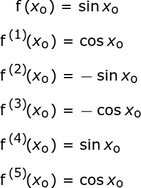#### Step 3: Substitution

The third and final step is to substitute the derivatives into the Taylor series general expression. This list below showing the different expressions with the substituted derivatives is the Taylor series for sin(x).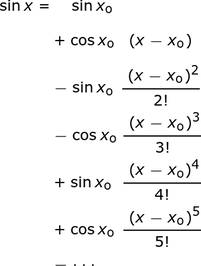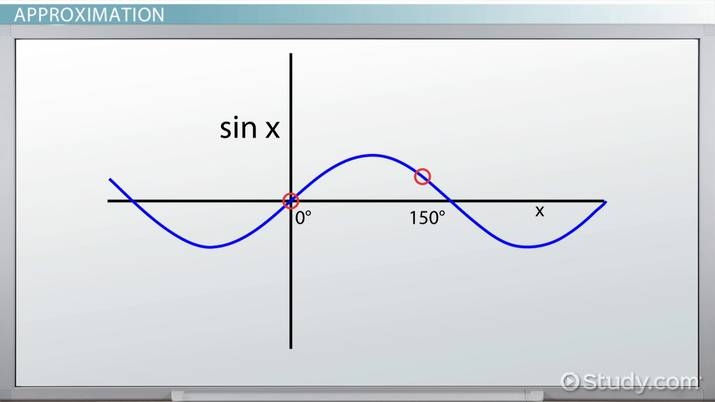An error occurred trying to load this video.

Try refreshing the page, or contact customer support.

Coming up next: Taylor Series for ln(1+x): How-to & Steps

### You're on a roll. Keep up the good work!

Replay
Your next lesson will play in 10 seconds
• 0:04 How to Find a Taylor Series
• 2:29 Approximation
• 5:32 Lesson Summary
Save Save

Want to watch this again later?

Timeline
Autoplay
Autoplay
Speed Speed

## Approximation

We can obtain a finite series approximation to f(x) by stopping the sum at some value of n. This is called a Taylor series polynomial or a truncated Taylor series. In the following examples, we keep the first 6 terms by summing up to n = 5.

The two points about which we will look at are 0o and 150o. First of all, the angle measures must be in radians. Thus, xo = 0o is 0 radians and xo = 150o = 150π/180 radians.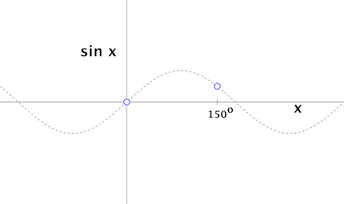Evaluating about xo = 0 for -π ≤ x ≤ π , we get: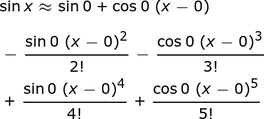Noting sin(0) = 0 and cos(0) = 1, we get: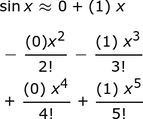Further simplifying, we get: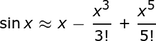These are the first three terms of the Maclaurin series for sin(x). A Maclaurin series is a specific type of Taylor series that is evaluated at xo = 0.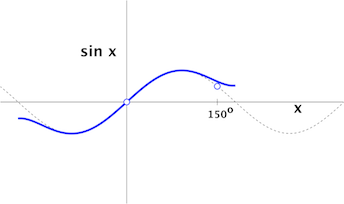The blue curve in the graph above is the series approximation. This curve is plotted with the sine curve for comparison. Impressively, there's a very good match for values close to x = 0, but the match is not very good at 150o.

To unlock this lesson you must be a Study.com Member.

### Register to view this lesson

Are you a student or a teacher?